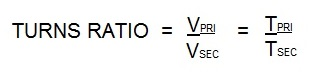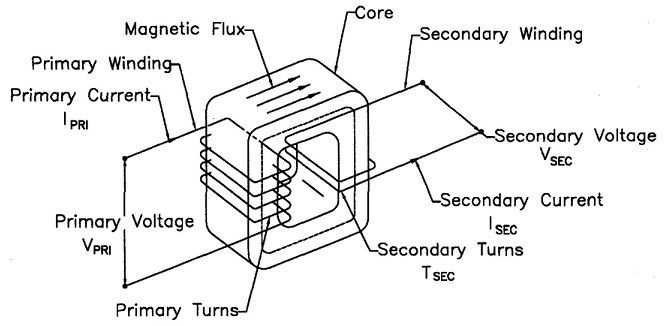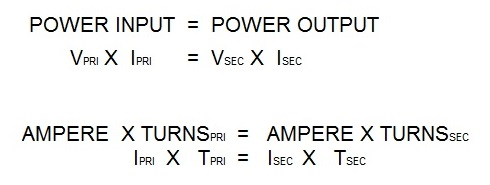# What is the turns ratio for a resistance welding transformer?

The transformer turns ratio in resistance welding reflects the number of secondary coil turns versus the number of primary coil turns. In AC transformers, there are many turns in the primary. The secondary has one turn. If there are 50 turns then the ratio is 50/1. Therefor the primary voltage will drop from 220 V and 100 amp input to 220/50 = 4.4 volts in the secondary. The equations for this are:Vpri = Voltage on the primary                     Tpri = Turns in the primary coil
Vsec= Voltage on the secondary                Tsec = Turns in the secondary coilPower into the transformer will equal power out of the transformer.Ipri = Primary amperage
Isec = Secondary amperage

Amperage in the secondary using the above formula is:
If the Ipri = 100 amperes
If the turns ratio = 50/1
Isec = (Ipri X Tpri)/ Tsec
Isec = 100 x 50/1
Isec = 5000 amperes

Reference: RWMA Manual Chapter 19

Roman Manufacturing Inc.

### Have a Question?

Do you have a question that is not covered in our knowledgebase? Do you have questions regarding the above article? Click here to ask the professor.